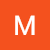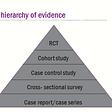# “This world of ours, ever growing smaller, must avoid becoming a community of dreadful fear and hate, and be, instead, a proud confederation of mutual trust and respect” — Dwight Eisenhower“Gen. Dwight D. Eisenhower at headquarters of the Pacific Air Command, U. S. Army (PACUSA), Manila, Philippines, May 1946” by J. Tewell is licensed with CC BY-NC 2.0

# “It is better to offer no excuse than a bad one” — George Washington

• Whataburger has more negative tweets than In-N-Out.
• The slope falls off quicker than In-N-Out’s, meaning less people were happy with it.
• So, according to Twitter, In-N-Out is the better burger joint.

# Nerdy Stuff for Nerdy People

• TextBlob, the NLP software mentioned earlier, converted each tweet into a number between -1 and 1, from negative to positive. The resultant data could then be plotted using Matplotlib with 50 histogram bars.
• Networkx, a network analysis software, takes all the tweets, and plots the tags between users as arrows. This was again plotted with Matplotlib, in the form of a node graph.
`"""Import required libraries:    twint             - For initial twitter scrape    os                - For removal of unwanted files    csv               - For interaction with csv files containing tweet data    networkx          - For network analysis    textblob          - For fine-grained sentimental analysis    time              - For pausing program temporarily    matplotlib.pyplot - For sentimental analysis and network analysis visualisation    numpy             - Backend for networkx"""import twint, os, csvfrom csv import readerfrom csv import writerimport networkx as nxfrom textblob import TextBlobfrom textblob import classifiersimport time as timport matplotlib.pyplot as pltimport numpy as np"""Configuration of basic libraries:    - Use basic plot style for matplotlib    - Set network analysis graphs to Directed graps (one node to another with an arrow)    - Initialise twint"""plt.style.use('seaborn-whitegrid')G=nx.DiGraph()c=twint.Config()"""Check if path exists, and removing it if so.In testing, required time delay to register as empty"""def REMOVE(path):    if os.path.exists(path):        os.remove(path)    t.sleep(2)    """Runs analysis of sentence with Natural Langauge Processor (NLP) software TextBlob.Returns both:    - polarity (float between -1 and 1, -1 being most negative, 1 being most positive).    - subjectivity (float between 0 and 1, 0 being objective, 1 being subjective)Subjectivity is not actually used in this program, but is there for optional later analysis"""def TextBlob_Sentiment(Extract):    sample=TextBlob(Extract)    polarity=(sample.sentiment.polarity)    subjectivity=(sample.sentiment.subjectivity)    return polarity, subjectivity"""Creates initial tweet database    - Removes output file in case of overlap    - Creates CSV file with 3 columns: username, tweet, and mentions    - Can be manually interrupted because of try command"""def TWITTER_SEARCH_KEYPHRASE(keyphrase,path):    REMOVE(path)    try: #Allows for manual discontinuation of search with keyboard interrupt        c.Search=("\"" + keyphrase + "\"")        c.Lang='en'        c.Limit=30000        c.Custom["tweet"] = ["username", "tweet", "mentions"]        c.Store_csv = True        c.Output = (path)        twint.run.Search(c)    except:        pass"""Creates a list of all values in a particular column:    - Skips header line    - Prints line count as confirmation of working    - Returns data list, and number of lines"""def CSV_Parse(path, column_number):    data=[]    with open(path) as csv_file:        csv_reader = csv.reader(csv_file, delimiter=',')        line_count = 0        for row in csv_reader:            if line_count == 0:                line_count += 1            else:                data.append(row[column_number])                line_count += 1    print(f'Processed {line_count} lines.')    return data, line_count"""Creates Carbon Copy CSV File:    - Takes some data from inital CSV file, and appends each row with Polarity and Subjectivity    - Labels Headers"""def CSV_Write(in_path, out_path, column_in, first_header):    REMOVE(out_path) #Prevents Overlap    with open(in_path,'r') as csvinput:        with open(out_path, 'w') as csvoutput:            writer = csv.writer(csvoutput)            for row in csv.reader(csvinput):                 if row == first_header: #If first cell contains header, appends correct headers                    writer.writerow([str(row), "Polarity", "Subjectivity"])                else:                    Sentiment = TextBlob_Sentiment(row[column_in])                    #Removes null readings caused by overly short tweets                     if (str(Sentiment)!='0.0' and str(Sentiment)!='0.0'):                         polarity = Sentiment                         subjectivity = Sentiment                         writer.writerow([str(row), polarity, subjectivity])"""Plots polarity histogram:    - Recieves polarity data from CSV_Parse function    - Plots total data points to histogram bars in ratio 50:1"""def PLOT(source):    data1=CSV_Parse(source, 1)    x=data1    fig, ax = plt.subplots()    Total_datapoints=int(data1)    Histogram_bars=int(50)    num_bins = (Histogram_bars)    n, bins, patches = ax.hist(x, num_bins, density=True)    ax.set_xlabel('Sentiment')    ax.set_ylabel('Arbitrary Units')    ax.set_title(r'Negative To Positive Tweet Sentiment')    fig.tight_layout()    plt.show()"""Cleans Edge values (@ tags):    - Removes [ symbols from the front    - Removes ] symbols from the back    - Remove Spaces    - Remove Apostrophes    - Create a list split by Commas"""def Network_Cleanup(Object):    Edge_RMB1=Object.replace('[','')    Edge_RMB2=Edge_RMB1.replace(']','')    Edge_No_Space=Edge_RMB2.replace(" ",'')    Edge_no_apost=Edge_No_Space.replace("\'","")    Edge_Final=Edge_no_apost.split(',')    return Edge_Final"""Adds edges to network:    - Reads from tweet database    - Removes any null values ("[]")    - Uses Cleanup function to clean edge values    - Creates relationships using the format (node, recieving node)    - Prints line count to confirm working script"""def Network_Graph_SingularCSVParse(path, node_column, edge_column):    with open(path) as csv_file:        csv_reader = csv.reader(csv_file, delimiter=',')        line_count = 0        for row in csv_reader:            if line_count == 0:                line_count += 1            else:                if row[edge_column]!="[]":                    Edge_With_Noise=row[edge_column]                    Edge=Network_Cleanup(Edge_With_Noise)                    for i in range(0,(len(Edge))):                        Node=row[node_column]                        G.add_edge(Node,Edge[i])                line_count += 1    print(f'Processed {line_count} lines.')"""Draws Network Graph:    - Calls CSV_Parse to add nodes    - Calls Network CSV Parse function to generate edges    - Print Layout Processing to indicate working (typically takes a while)    - Generate Fruchterman-Reingold force-directed algorithm (sprint_layout)    - When processed print "Done" to indicate loading (also normally takes a while)    - Show graph"""def Network_Graph(path, node_column, edge_column, label, title):    Node_Parser=CSV_Parse(path, node_column)    Node_Values=Node_Parser    G.add_nodes_from(Node_Values)    Network_Graph_SingularCSVParse(path, node_column, edge_column)    print("Layout Processing")    pos=nx.spring_layout(G, k=0.1, iterations=50)    plt.figure(num=title,figsize=(133,100), clear=True)    nx.draw_networkx(G, pos=pos,edge_color="black", linewidths=0.1, arrowsize=2, node_size=10, with_labels=label, alpha=0.7, width=0.7, font_size=12)    print("Done")    plt.show()    """Trigger start of program:    - Call twitter scrape with inputted keyphrase    - If sentimental analysis, run required functions    - If network analysis, run required functions"""def Initiate(keyphrase, main_path, out_path, output, label):    TWITTER_SEARCH_KEYPHRASE(keyphrase, main_path)    if output==1:        CSV_Write(main_path, out_path, 1, "username")        PLOT(out_path)    else:        Network_Graph(main_path, 0, 2, label, str(keyphrase + " Network"))       """Allow interactive input to the script:    - Keyphrase    - Optional Central File Name    - Optional Carbon-Copy File Name    - Desired Output (sentimental analysis or network analysis)        - If network analysis, desired node labels"""def Input():    print("Please Enter Searchable Keyphrase:")    Sorted1=False    while Sorted1==False:        try:            keyphrase=str(input())            Sorted1=True        except:            print("Invalid Input")    print("Please Enter Centralised File Name (default:tweets.csv)")    Sorted2=False    while Sorted2==False:        try:            main_path=str(input())            if main_path=="":                main_path="tweets.csv"            Sorted2=True        except:            print("Invalid Input")    print("Please Enter Working File Name (default:out.csv)")    Sorted3=False    while Sorted3==False:        try:            out_path=str(input())            if out_path=="":                out_path="out.csv"            Sorted3=True        except:            print("Invalid Input")    print("Please Enter Desired Output (1 = Sentimental, 2 = Network):")    Sorted4=False    while Sorted4==False:        try:            output=int(input())            if output==1 or output==2:                Sorted4=True            else:                print("Invalid Input")        except:            print("Invalid Input")    label=False    if output==2:        print("Do you want to have node labels? y/n:")           Sorted5=False        while Sorted5==False:            try:                label_in=str(input())                if label_in=='y':                    label=True                    Sorted5=True                if label_in=='n':                    label=False                    Sorted5=True                if label_in!='y' and label_in!='n':                    print("Invalid Input")            except:                print("Invalid Input")    Initiate(keyphrase, main_path, out_path, output, label) """Start Program"""Input()`

--

--

--

## More from Dan

Love podcasts or audiobooks? Learn on the go with our new app.

## Dr. Robin Murray | RN, MSN, DNP## Repeached: Donald J. Trump Makes History## A Message to a Friend; The Past, Present, and Future of the Police## Howard, run for President## President Trump: Cancel Student Loans by Executive Order.## “God’s Master Plan” 3–11–2022## Kicking a dream that became an addiction## Genevieve the cryptanalysis genius## Constructing a critical argument## Heartstopper’s Nick Nelson is the Queer Archetype we’d failed to imagine.## The Artist’s Stance — by Lib Briscoe